Two Views of the Pythagorean Theorem

The familiar Pythagorean theorem states that if a right triangle has legs a and b and hypotenuse c, then a2 + b2 = c2. In geometric terms, we can think of a2, b2, and c2 as the areas of three squares, one on each side of the triangle. In this view, the theorem says the area of the square on the hypotenuse is equal to the sum of the areas of the squares on the other two sides. Although this is one of the most useful facts in analytic geometry, and just about everyone has heard of it, not everyone knows a proof. Do you?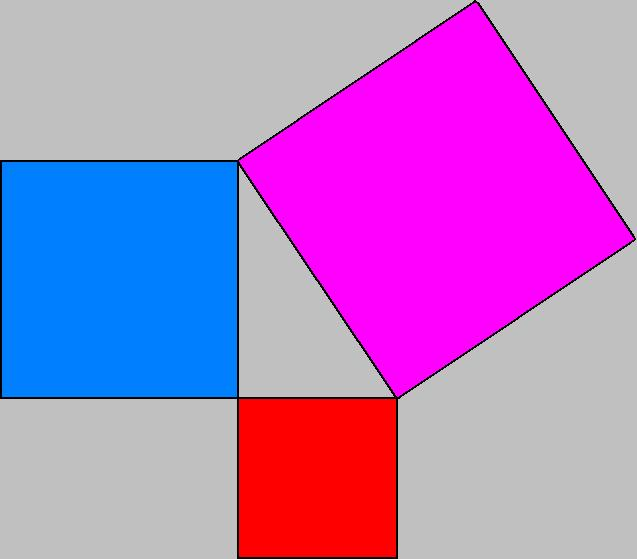Pythagorean Theorem: Area of the purple square equals the sum of the areas of blue and red squares.

It turns out that there are dozens of known proofs for the Pythagorean Theorem. One proof was even given by a president of the United States! With all of these proofs to choose from, everyone should know at least one favorite proof. In this article I will share two of my personal favorites.

The first proof   begins with an arbitrary right triangle, and assembles four identical copies to make a large square, as shown below.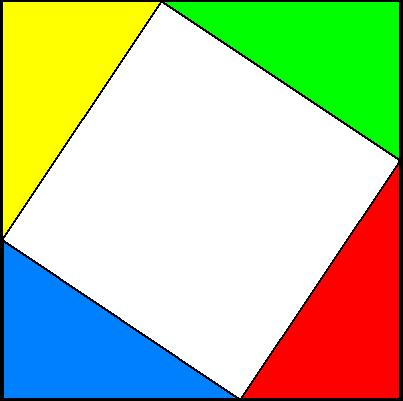Four copies of the triangle arranged in a square.

If the short leg of each triangle is a, the longer leg b, and the hypotenuse c, then we can put the four triangles in to the corners of a square of side a+b. The two triangles along each side of the large square just cover that side, meeting in a single point. Thus, the white part of the figure is a quadrilateral with each of its sides equal to c. In fact, it is actually a square. One way to see this is by symmetry -- each side of the figure is identical to every other side, so the four corner angles of the white quadrilateral all have to be equal. And that can only be true if they are all right angles. Another way to see the same thing uses the fact that the two acute angles in any right triangle add up to 90 degrees. Now at each corner of the white quadrilateral we have the two different acute angles of the original right triangle. Since these add to 90 degrees, the white angle separating them must also be 90 degrees. Either way you look at it, the conclusion is the same: when four identical copies of the right triangle are arranged in a square of side a+b, they form a square of side c in the middle of the figure.

This leads to a proof of the Pythagorean theorem by sliding the colored triangles around in the large square. As long as the colored triangles don't overlap and remain inside the boundaries of the large square, the remaining white part must always take up the same amount of area. Originally, that area is c2, given by a square of side c. But with an appropriate rearrangement, you can see that the white area also fills up two smaller squares, one of side a and one of side b. This shows that a2 + b2 = c2, and so proves the theorem. You can see an animated display of the moving triangles below.

The second proof
is one I read in George Polya's Analogy and Induction,  a classic book on mathematical thinking.  It begins by observing that the squares on the sides of the right triangle can be replaced with any other figures as long as similar figures are used on each side.  For example, replace each square with a semi-circle, or a similar isoceles triangle, as shown below.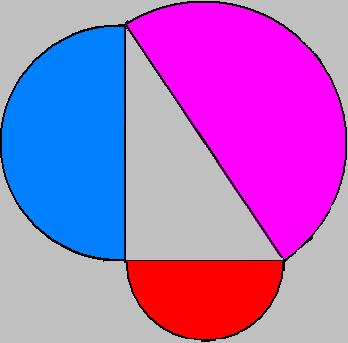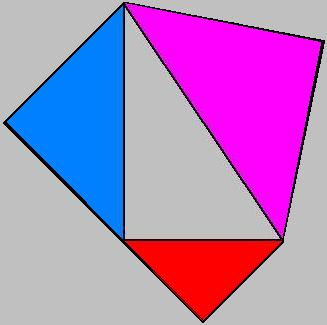Replace squares with similar figures on each side of the right triangle.

The thing about similar figures is that they can be made congruent by a simple magnification or contraction of scale.  For example, in the first figure, there is a semi-circle on each side of the triangle.  The red semicircle has diameter a, whereas the blue semicircle has diameter b.  That means that expanding the red semi-circle by a factor of b/a will make it congruent to the blue triangle.  And exactly the same is true of the red and blue isosceles triangles in the second figure.  Indeed, put any figure whatsoever on each side of the triangle, always using similar figures mind, and the following proportions will hold:  the blue figure will be a  b/a magnification of the red, and the purple will be a c/a magnification of the red.

Now, what happens to the area of a figure when you magnify it by a factor of t?  The answer is, it increases by a factor of t2. After all, the very definition of area has to do with filling up a figure with tiny squares, and taking a limit as the size of the squares goes to 0.  And clearly for a square, if you stretch or shrink each side by a factor of t, then the area will increase or decrease by a factor of t2.  Since this will be true for all the little squares filling up a figure, it will also be true of the overall area of the figure.

So now, suppose that we put similar figures on each side of the triangle, and that the red figure has area A.  Then the blue figure will have area (b/a)2A  and the purple will have area (c/a)2A.   Now the red area plus the blue area will equal the purple area if and only if   A + (b/a)2A = (c/a)2A, and that is equivalent to a2 + b2 = c2.  So, if the areas add up correctly for a particular figure (like squares, or semi-circles) then they have to add up for every figure.

With that in mind, consider the figure below, in which the original triangle is shown, with a perpendicular line drawn from the right angle to the hypotenuse.  The red and blue triangles are each similar to the original triangle.  But look: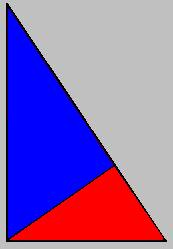Triangle with altitude drawn to the hypotenuse.

the red triangle has been drawn with its hypotenuse on the shorter leg of the triangle; the blue triangle is a similar figure drawn with its hypotenuse on the longer leg of the triangle.  Meanwhile, the entire triangle is again similar and can be considered to be drawn with its hypotenues on  --- its hypotenuse.  Since the blue and red figures clearly fill up the entire triangle, that proves the Pythagorean theorem!

A large number of proofs of the Pythagorean theorem, including several with dynamic geometry animations, are presented at http://www.cut-the-knot.org/pythagoras/index.shtml. Links to several animated proofs can also be found at http://hlm.hkcampus.net/~hlm-ctw/java.htm#pythagorean.